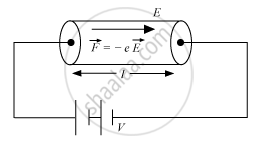Share

# Define the Term 'Drift Velocity' of Charge Carriers in a Conductor. Obtain the Expression for the Current Density in Terms of Relaxation Time ? - Physics

ConceptElectric Current

#### Question

Define the term 'drift velocity' of charge carriers in a conductor. Obtain the expression for the current density in terms of relaxation time ?

#### Solution

Drift velocity: It is the velocity with which free electrons get drifted towards the positive terminal under the effect of the applied electric field.

Free electrons are in continuous random motion. They undergo change in direction at each collision and the thermal velocities are randomly distributed in all directions.

∴ Average thermal velocity,u =(u_1+u_2+ ...+u_n)/n = 0 ....... (1)The electric field E exerts an electrostatic force −Ee.

Acceleration of each electron,veca = (-evecE)/m      ....... (2)

where,

m = mass of an electron

e = charge on an electron

(vecv_1+vecv_2+ ...+vecv_n)/n

vecv_d = (vecu_1+vecatau_1)+(vecu_2+vecatau_2)+ ........+(vecu_n+vecatau_n)

where,

vecu_1 ,vecu_2 ->thermal velocities of the electrons

vecatau_1 ,vecatau_2 -> velocity acquired by electrons

tau_1 ,tau_2 ->  time elapsed after the collision

vecv = (vecu_1 +vecu_2 +....vecu_n)/n + veca(tau_1+tau_2+.....+tau_n)/n

Since vecv = (vecu_1 +vecu_2 +....vecu_n)/n = 0, we get:

∴ vd τ, where, veca(tau_1+tau_2+tau_3+.....+tau_n)/n

is the average time elapsed.

Substituting the value of from equation (2), we have:

vecv_d = (-evecE)/m tau  ....... (4)

Is there an error in this question or solution?

#### Video TutorialsVIEW ALL 

Solution Define the Term 'Drift Velocity' of Charge Carriers in a Conductor. Obtain the Expression for the Current Density in Terms of Relaxation Time ? Concept: Electric Current.
S